# Difference between revisions of "Heavy-light decomposition"

The heavy-light (H-L) decomposition of a rooted tree is a method of partitioning of the vertices of the tree into disjoint paths (all vertices have degree two, except the endpoints of a path, with degree one) that gives important asymptotic time bounds for certain problems involving trees. It appears to have been introduced in passing in Sleator and Tarjan's analysis of the performance of the link-cut tree data structure.

## Definition

The heavy-light decomposition of a tree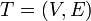$T=(V,E)$ is a coloring of the tree's edges. Each edge is either heavy or light. To determine which, consider the edge's two endpoints: one is closer to the root, and one is further away. If the size of the subtree rooted at the latter is more than half that of the subtree rooted at the former, the edge is heavy. Otherwise, it is light.

## Properties

Denote the size of a subtree rooted at vertex$v$ as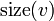$\operatorname{size}(v)$.

Suppose that a vertex$v$ has two children$u$ and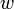$w$ and the edges$v$-$u$ and$v$-$w$ are both heavy. Then,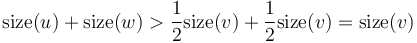$\operatorname{size}(u) + \operatorname{size}(w) > \frac{1}{2}\operatorname{size}(v)+\frac{1}{2}\operatorname{size}(v) = \operatorname{size}(v)$. This is a contradiction, since we know that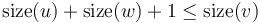$\operatorname{size}(u) + \operatorname{size}(w) + 1 \le \operatorname{size}(v)$. ($v$, of course, may have more than two children.) We conclude that there may be at most one heavy edge of all the edges joining any given vertex to its children.

At most two edges incident upon a given vertex may then be heavy: the one joining it to its parent, and at most one joining it to a child. Consider the subgraph of the tree in which all light edges are removed. Then, all resulting connected components are paths (although some contain only one vertex and no edges at all) and two neighboring vertices' heights differ by one. We conclude that the heavy edges, along with the vertices upon which they are incident, partition the tree into disjoint paths, each of which is part of some path from the root to a leaf.

Suppose a tree contains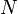$N$ vertices. If we follow a light edge from the root, the subtree rooted at the resulting vertex has size at most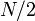$N/2$; if we repeat this, we reach a vertex with subtree size at most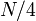$N/4$, and so on. It follows that the number of light edges on any path from root to leaf is at most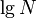$\lg N$.

## Applications

The utility of the H-L decomposition lies in that problems involving paths between nodes can often be solved more efficiently by "skipping over" heavy paths rather than considering each edge in the path individually. This is because long paths in trees that are very poorly balanced tend to consist mostly of heavy edges.

To "skip" from any node in the tree to the root:

while current_node ≠ root
if color(current_node,parent(current_node)) = light
current_node = parent(current_node)
else
current_node = skip(current_node)


In the above, color(u,v) represents the color (heavy or light) of the edge from u to v, parent(v) represents the parent of a node v, and skip(v) represents the parent of the highest (i.e., least deep) node that is located on the same heavy path as node v. (It is so named because it allows us to "skip" through heavy paths.) In order for the latter to be implemented efficiently, every node whose parent link is heavy must be augmented with a pointer to the result of the skip function, but that is trivial to achieve.

What is the running time of this fragment of code? There are at most a logarithmic number of light edges on the path, each of which takes constant time to traverse. There are also at most a logarithmic number of heavy subpaths, since between each pair of adjacent heavy paths lies a light path. We spend at most a constant amount of time on each of these, so overall this "skipping" operation runs in logarithmic time.

This might not seem like much, but let's see how it can be used to solve a simple problem.

### Dynamic distance query

Consider the following problem: A weighted, rooted tree is given followed by a large number of queries and modifications interspersed with each other. A query asks for the distance between a given pair of nodes and a modification changes the weight of a specified edge to a new (specified) value. How can the queries and modifications be performed efficiently?

First of all, we notice that the distance between nodes$u$ and$v$ is given by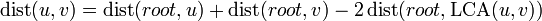$\operatorname{dist}(u,v) = \operatorname{dist}(root,u) + \operatorname{dist}(root,v) - 2\,\operatorname{dist}(root,\operatorname{LCA}(u,v))$. There are a number of techniques for efficiently answering lowest common ancestor queries, so if we can efficiently solve queries of a node's distance from the root, a solution to this problem follows trivially.

To solve this simpler problem of querying the distance from the root to a given node, we augment our walk procedure as follows: when following a light edge, simply add its distance to a running total; when following a heavy path, add all the distances along the edges traversed to the running total (as well as the distance along the light edge at the top, if any). The latter can be performed efficiently, along with modifications, if each heavy path is augmented with a data structure such as a segment tree or binary indexed tree (in which the individual array elements correspond to the weights of the edges of the heavy path).

This gives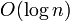$O(\log n)$ time for queries, since it consists of two "skips" and a LCA query, all of which take logarithmic time or better. It might seem that we have to ascend logarithmically many heavy paths each of which takes logarithmic time, but this is not the case; only the first one, if we start out on it, has to take logarithmic time to query; for the others, which we skip over in their entirety, we can just look up a variable indicating the sum of all weights on that path, which can be updated in constant time when any edge on that path is updated. An update, merely an update to the underlying array structure, takes$O(\log n)$ time also.